# Apply a function to each row or column in Dataframe using pandas.apply()

• Difficulty Level : Medium
• Last Updated : 19 Jul, 2021

There are different ways to apply a function to each row or column in DataFrame. We will learn about various ways in this post. Let’s create a small dataframe first and see that.

## Python3

 `# import pandas and numpy library``import` `pandas as pd``import` `numpy as np`` ` `# list of tuples``matrix ``=` `[(``1``,``2``,``3``,``4``),``          ``(``5``,``6``,``7``,``8``,),``          ``(``9``,``10``,``11``,``12``),``          ``(``13``,``14``,``15``,``16``)``         ``]`` ` `# Create a Dataframe object``df ``=` `pd.DataFrame(matrix, columns ``=` `list``(``'abcd'``))`` ` `# Output``df`

Output :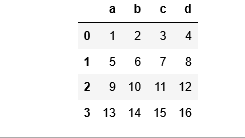Method 1: Applying lambda function to each row/column.
Example 1: For Column

## Python3

 `# import pandas and numpy library``import` `pandas as pd``import` `numpy as np` `# list of tuples``matrix ``=` `[(``1``,``2``,``3``,``4``),``          ``(``5``,``6``,``7``,``8``,),``          ``(``9``,``10``,``11``,``12``),``          ``(``13``,``14``,``15``,``16``)``         ``]` `# Create a Dataframe object``df ``=` `pd.DataFrame(matrix, columns ``=` `list``(``'abcd'``))` `# Applying a lambda function to each``# column which will add 10 to the value``new_df ``=` `df.``apply``(``lambda` `x : x ``+` `10``)` `# Output``new_df`

Output :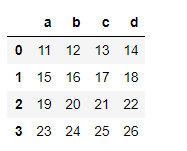Example 2: For Row

## Python3

 `# import pandas and numpy library``import` `pandas as pd``import` `numpy as np` `# list of tuples``matrix ``=` `[(``1``,``2``,``3``,``4``),``          ``(``5``,``6``,``7``,``8``,),``          ``(``9``,``10``,``11``,``12``),``          ``(``13``,``14``,``15``,``16``)``         ``]` `# Creating a Dataframe object``df ``=` `pd.DataFrame(matrix, columns ``=` `list``(``'abcd'``))` `# Applying a lambda function to each``# row which will add 5 to the value``new_df ``=` `df.``apply``(``lambda` `x: x ``+` `5``, axis ``=` `1``)` `# Output``new_df`

Output :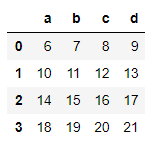Method 2: Applying user defined function to each row/column
Example 1: For Column

## Python3

 `# function to returns x*x``def` `squareData(x):``    ``return` `x ``*` `x` `# import pandas and numpy packages``import` `pandas as pd``import` `numpy as np` `# list of tuples``matrix ``=` `[(``1``,``2``,``3``,``4``),``          ``(``5``,``6``,``7``,``8``,),``          ``(``9``,``10``,``11``,``12``),``          ``(``13``,``14``,``15``,``16``)``         ``]` `# Creating a Dataframe object``df ``=` `pd.DataFrame(matrix, columns ``=` `list``(``'abcd'``))` `# Applying a user defined function to``# each column that will square the given``# value``new_df ``=` `df.``apply``(squareData)` `# Output``new_df`

Output :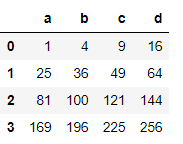Example 2: For Row

## Python3

 `# function to returns x*X``def` `squareData(x):``    ``return` `x ``*` `x` `# import pandas and numpy library``import` `pandas as pd``import` `numpy as np` `# List of tuples``matrix ``=` `[(``1``,``2``,``3``,``4``),``          ``(``5``,``6``,``7``,``8``,),``          ``(``9``,``10``,``11``,``12``),``          ``(``13``,``14``,``15``,``16``)``         ``]` `# Creating a Dataframe object``df ``=` `pd.DataFrame(matrix, columns ``=` `list``(``'abcd'``))` `# Applying a user defined function``# to each row that will square the given value``new_df ``=` `df.``apply``(squareData, axis ``=` `1``)` `# Output``new_df`

Output :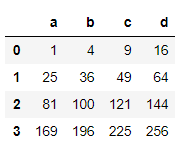In the above examples, we saw how a user defined function is applied to each row and column. We can also apply user defined functions which take two arguments.

Example 1: For Column

## Python3

 `# function to returns x+y``def` `addData(x, y):``    ``return` `x ``+` `y` `# import pandas and numpy library``import` `pandas as pd``import` `numpy as np` `# list of tuples``matrix ``=` `[(``1``,``2``,``3``,``4``),``          ``(``5``,``6``,``7``,``8``,),``          ``(``9``,``10``,``11``,``12``),``          ``(``13``,``14``,``15``,``16``)``         ``]` `# Creating a Dataframe object``df ``=` `pd.DataFrame(matrix, columns ``=` `list``(``'abcd'``))` `# Applying a user defined function to each``# column which will add value in each``# column by given number``new_df ``=` `df.``apply``(addData, args ``=` `[``1``])` `# Output``print``(new_df)`

Output: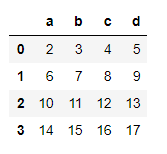Example 2: For Row

## Python3

 `# function to returns x+y``def` `addData(x, y):``    ``return` `x ``+` `y` `# import pandas and numpy library``import` `pandas as pd``import` `numpy as np` `# List of tuples``matrix ``=` `[(``1``,``2``,``3``,``4``),``          ``(``5``,``6``,``7``,``8``,),``          ``(``9``,``10``,``11``,``12``),``          ``(``13``,``14``,``15``,``16``)``         ``]` `# Creating a Dataframe object``df ``=` `pd.DataFrame(matrix, columns ``=` `list``(``'abcd'``))` `# Applying a user defined function to each``# row which will add value in each row by``# given number``new_df ``=` `df.``apply``(addData, axis ``=` `1``,``                    ``args ``=` `[``3``])` `# Output``new_df`

Output :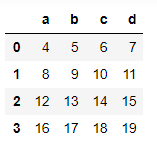Method 3: Applying numpy function to each row/column
Example 1: For Column

## Python3

 `# import pandas and numpy library``import` `pandas as pd``import` `numpy as np` `# list of tuples``matrix ``=` `[(``1``,``2``,``3``,``4``),``          ``(``5``,``6``,``7``,``8``,),``          ``(``9``,``10``,``11``,``12``),``          ``(``13``,``14``,``15``,``16``)``         ``]` `# Creating a dataframe object``df ``=` `pd.DataFrame(matrix, columns ``=` `list``(``'abcd'``))` `# Applying a numpy function to each``# column by squaring each value``new_df ``=` `df.``apply``(np.square)` `# Output``new_df`

Output :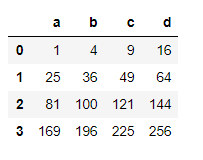Example 2: For Row

## Python3

 `# import pandas and numpy library``import` `pandas as pd``import` `numpy as np` `# List of tuples``matrix ``=` `[(``1``,``2``,``3``,``4``),``          ``(``5``,``6``,``7``,``8``,),``          ``(``9``,``10``,``11``,``12``),``          ``(``13``,``14``,``15``,``16``)``         ``]` `# Creating a dataframe object``df ``=` `pd.DataFrame(matrix, columns ``=` `list``(``'abcd'``))` `# Apply a numpy function to each row``# to find square root of each value``new_df ``=` `df.``apply``(np.sqrt, axis ``=` `1``)` `# Output``new_df`

Output :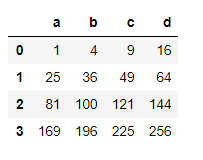Method 4: Applying a Reducing function to each row/column
A Reducing function will take row or column as series and returns either a series of same size as that of input row/column or it will return a single variable depending upon the function we use.

Example 1: For Column

## Python3

 `# import pandas and numpy library``import` `pandas as pd``import` `numpy as np` `# List of tuples``matrix ``=` `[(``1``,``2``,``3``,``4``),``          ``(``5``,``6``,``7``,``8``,),``          ``(``9``,``10``,``11``,``12``),``          ``(``13``,``14``,``15``,``16``)``         ``]` `# Creating a Dataframe object``df ``=` `pd.DataFrame(matrix, columns ``=` `list``(``'abcd'``))` `# Applying a numpy function to get the sum``# of all values in each column``new_df ``=` `df.``apply``(np.``sum``)` `# Output``new_df`

Output :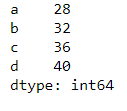Example 2: For Row

## Python3

 `# import pandas and numpy library``import` `pandas as pd``import` `numpy as np` `# List of tuples``matrix ``=` `[(``1``,``2``,``3``,``4``),``          ``(``5``,``6``,``7``,``8``,),``          ``(``9``,``10``,``11``,``12``),``          ``(``13``,``14``,``15``,``16``)``         ``]` `# Creating a Dataframe object``df ``=` `pd.DataFrame(matrix, columns ``=` `list``(``'abcd'``))` `# Applying a numpy function to get t``# he sum of all values in each row``new_df ``=` `df.``apply``(np.``sum``, axis ``=` `1``)` `# Output``new_df`

Output :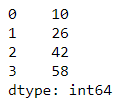My Personal Notes arrow_drop_up